## Introduction¶

Hypothesis Testing so important for Data Scientist, because you should know how to check distributions and relations between data.

In this tutorial, we will learn statistical hypothesis tests that you may need to use in your machine learning career. And after completing this tutorial, you will know how testing hypothesis for:

• types of hypothesis tests
• normality checking
• relationships checking between variables
• differences between samples
• how to interpret the test result
• how to implement the test using the Python

In this tutorial, you discovered the key statistical hypothesis tests that you may need to use in a machine learning project.

Specifically, you learned:

The types of tests to use in different circumstances, such as normality checking, relationships between variables, and differences between samples. The key assumptions for each test and how to interpret the test result. How to implement the test using the Python

## Interpretation of a Test¶

Before you can apply the statistical tests, you must know how to interpret the results.

Each test will return at least two things:

• Statistic: A quantity calculated by the test that can be interpreted in the context of the test via comparing it to critical values from the distribution of the test statistic.
• $p-value$: Used to interpret the test, in first case wheher the sample will drawn from a Gaussian distribution.

Each test calculates a test-specific statistic. This statistic can aid in the interpretation of the result, although it may require a deeper proficiency with statistics and a deeper knowledge of the specific statistical test. Instead, the p-value can be used to quickly and accurately interpret the statistic in practical applications.

At first part we'll be testing data that the sample was drawn from a Gaussian distribution. Technically this is called the null hypothesis, or H0. A threshold level is chosen called alpha, typically 5% (or 0.05), that is used to interpret the p-value. Interpret the p value as follows:

• $p <= alpha$: reject H0, not normal.
• $p > alpha$: fail to reject H0, normal.

This means that, in general, we are seeking results with a larger p-value to confirm that our sample was likely drawn from a Gaussian distribution.

A result above 5% DOESN'T MEAN that the null hypothesis is true. It means that it is very likely true given available evidence. The p-value is not the probability of the data fitting a Gaussian distribution; it can be thought of as a value that helps us interpret the statistical test.

## Generating Test Data ¶

In :
import matplotlib.pyplot as plt
import numpy as np
import seaborn as sns

In :
normal = np.random.standard_normal(
1000
)  # Draw random samples from a normal (Gaussian) distribution.
uniform = np.random.uniform(size=1000)
log_normal = np.random.lognormal(size=1000)

In :
sns.distplot(log_normal)
sns.distplot(normal);

Out:
<matplotlib.axes._subplots.AxesSubplot at 0x7ff3ccd8a470>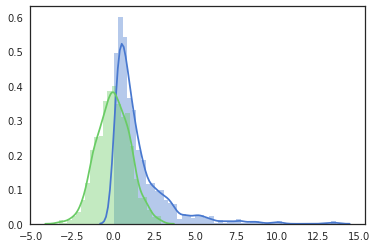In :
sns.distplot(uniform);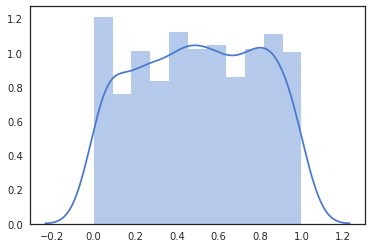## Statistical Hypothesis Tests¶

### Normality Tests ¶

This section lists statistical tests that you can use to check if your data has a Gaussian distribution.

### Shapiro-Wilk Test ¶

Tests whether a data sample has a Gaussian distribution(Normal).

Assumptions

Observations in each sample are independent and identically distributed (iid).
Interpretation

• H0: the sample has a Gaussian distribution.
• H1: the sample does not have a Gaussian distribution.

Returns the test statistic and the p-value.

In :
from scipy.stats import shapiro

shapiro(normal), shapiro(log_normal)

Out:
((0.9985584020614624, 0.5931937098503113),
(0.6438806056976318, 2.709410580772034e-41))

### D’Agostino’s $K^2$ Test ¶

The D’Agostino’s K^2 test calculates summary statistics from the data, namely kurtosis and skewness, to determine if the data distribution departs from the normal distribution, named for Ralph D’Agostino.

• Skew is a quantification of how much a distribution is pushed left or right, a measure of asymmetry in the distribution.
• Kurtosis quantifies how much of the distribution is in the tail. It is a simple and commonly used statistical test for normality.

The D’Agostino’s K^2 test is available via the normaltest() SciPy function and returns the test statistic and the p-value.

The complete example of the D’Agostino’s K^2 test on the dataset is listed below.

In :
from scipy.stats import normaltest

stat, p = normaltest(normal)
print("Statistics=%.3f, p=%.3f" % (stat, p))

alpha = 0.05
if p > alpha:
print("Sample looks Gaussian (fail to reject H0)")
else:
print("Sample does not look Gaussian (reject H0)")

Statistics=0.829, p=0.661
Sample looks Gaussian (fail to reject H0)

In :
from scipy.stats import normaltest

stat, p = normaltest(log_normal)
print("Statistics=%.3f, p=%.3f" % (stat, p))

alpha = 0.05
if p > alpha:
print("Sample looks Gaussian (fail to reject H0)")
else:
print("Sample does not look Gaussian (reject H0)")

Statistics=787.383, p=0.000
Sample does not look Gaussian (reject H0)


### Anderson-Darling Test ¶

• H0: the sample has a Gaussian distribution.
• H1: the sample does not have a Gaussian distribution.
In :
from scipy.stats import anderson

result = anderson(normal)
print("Statistic: %.3f" % result.statistic)
p = 0
for i in range(len(result.critical_values)):
sl, cv = result.significance_level[i], result.critical_values[i]
if result.statistic < result.critical_values[i]:
print("%.3f: %.3f, data looks normal (fail to reject H0)" % (sl, cv))
else:
print("%.3f: %.3f, data does not look normal (reject H0)" % (sl, cv))

Statistic: 0.378
15.000: 0.574, data looks normal (fail to reject H0)
10.000: 0.653, data looks normal (fail to reject H0)
5.000: 0.784, data looks normal (fail to reject H0)
2.500: 0.914, data looks normal (fail to reject H0)
1.000: 1.088, data looks normal (fail to reject H0)

In :
from scipy.stats import anderson

result = anderson(uniform)
print("Statistic: %.3f" % result.statistic)
p = 0
for i in range(len(result.critical_values)):
sl, cv = result.significance_level[i], result.critical_values[i]
if result.statistic < result.critical_values[i]:
print("%.3f: %.3f, data looks normal (fail to reject H0)" % (sl, cv))
else:
print("%.3f: %.3f, data does not look normal (reject H0)" % (sl, cv))

Statistic: 12.495
15.000: 0.574, data does not look normal (reject H0)
10.000: 0.653, data does not look normal (reject H0)
5.000: 0.784, data does not look normal (reject H0)
2.500: 0.914, data does not look normal (reject H0)
1.000: 1.088, data does not look normal (reject H0)


### Visual Normality Checks ¶

#### Histogram Plot ¶

A simple and commonly used plot to quickly check the distribution of a sample of data is the histogram. A sample of data has a Gaussian distribution of the histogram plot, showing the familiar bell shape.

In :
# Histogram Plot Gaussian distribution
sns.distplot(normal);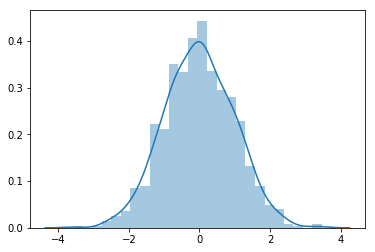In :
# Histogram Plot Uniform distribution
sns.distplot(uniform);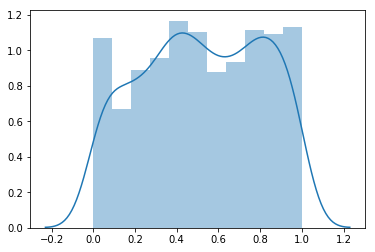#### Quantile-Quantile Plot ¶

Another popular plot for checking the distribution of a data sample is the quantile-quantile plot, Q-Q plot, or QQ plot for short.

This plot generates its own sample of the idealized distribution that we are comparing with, in this case the Gaussian distribution. The idealized samples are divided into groups (e.g. 5), called quantiles. Each data point in the sample is paired with a similar member from the idealized distribution at the same cumulative distribution.

The resulting points are plotted as a scatter plot with the idealized value on the x-axis and the data sample on the y-axis.

A perfect match for the distribution will be shown by a line of dots on a 45-degree angle from the bottom left of the plot to the top right. Often a line is drawn on the plot to help make this expectation clear. Deviations by the dots from the line shows a deviation from the expected distribution.

In :
import statsmodels.api as sm
from matplotlib import pyplot as plt

fig = sm.qqplot(normal, line="s")
plt.show()  # Gaussian distribution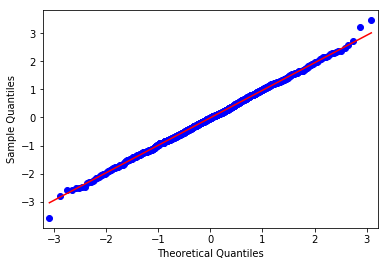In :
fig = sm.qqplot(log_normal, line="s")
plt.show()  # Log Normal distribution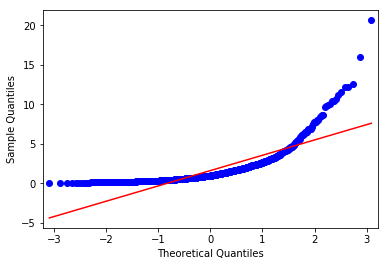## Correlation Tests ¶

This section lists statistical tests that you can use to check if two samples are related.

In :
x = np.random.normal(0, 1, 1000)
y = (3 * x) - np.random.normal(0, 2, 1000)


### Pearson’s Correlation Coefficient ¶

Tests whether two samples have a linear relationship.

• H0: the two samples are independent.
• H1: there is a dependency between the samples.
In :
from scipy.stats import pearsonr

corr, p = pearsonr(x, y)
corr, p

Out:
(0.8207236708707059, 7.2042131600280555e-245)

### Spearman’s Rank Correlation ¶

Tests whether two samples have a monotonic relationship. Observations in each sample can be ranked.

• H0: the two samples are independent.
• H1: there is a dependency between the samples.
In :
from scipy.stats import spearmanr

corr, p = spearmanr(x, y)
corr, p

Out:
(0.8076902436902438, 3.2834508833351045e-231)

### Kendall’s Rank Correlation ¶

Tests whether two samples have a monotonic relationship. Like in previous

In :
from scipy.stats import kendalltau

corr, p = kendalltau(x, y)
corr, p

Out:
(0.6135895895895896, 1.3595289338753751e-185)

## Parametric Statistical Hypothesis Tests ¶

This section lists statistical tests that you can use to compare data samples. Parametric statistical methods often mean those methods that assume the data samples have a Gaussian distribution. Parametric statistical significance tests that quantify the difference between the means of two or more samples of data.

For parametric statistical tests assume that a data sample was drawn from a specific population distribution.

They often refer to statistical tests that assume the Gaussian distribution. Because it is so common for data to fit this distribution, parametric statistical methods are more commonly used.

A typical question we may have about two or more samples of data is whether they have the same distribution. Parametric statistical significance tests are those statistical methods that assume data comes from the same Gaussian distribution, that is a data distribution with the same mean and standard deviation: the parameters of the distribution.

The p-value can be thought of as the probability of observing the two data samples given the base assumption (null hypothesis) that the two samples were drawn from a population with the same distribution.

p <= alpha: reject null hypothesis, different distribution.
p > alpha: fail to reject null hypothesis, same distribution

In :
x1 = 3 * np.random.randn(1000) + 20
x2 = 3 * np.random.randn(1000) + 21
print("x1: mean=%.3f stdv=%.3f" % (np.mean(x1), np.std(x1)))
print("x2: mean=%.3f stdv=%.3f" % (np.mean(x2), np.std(x2)))
sns.distplot(x1)
sns.distplot(x2);

x1: mean=20.042 stdv=3.046
x2: mean=21.002 stdv=2.913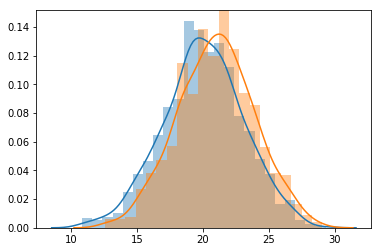### Student’s t-test ¶

Tests whether the means of two independent samples are significantly different. One of the most commonly used t tests is the independent samples t test. You use this test when you want to compare the means of two independent samples on a given variable

Assumptions

• Observations in each sample are independent and identically distributed (iid).
• Observations in each sample are normally distributed.
• Observations in each sample have the same variance.

Interpretation

• H0: the means of the samples are equal.
• H1: the means of the samples are unequal.
In :
from scipy.stats import ttest_ind

stat, p = ttest_ind(x1, x2)
print("P value:", p)
alpha = 0.05
if p > alpha:
print("Same distributions (fail to reject H0)")
else:
print("Different distributions (reject H0)")

P value: 8.810726433096305e-13
Different distributions (reject H0)


The interpretation of the statistic finds that the sample means are different, with a significance of at least 5%.

### Paired Student’s t-test ¶

Tests whether the means of two paired samples are significantly different. The paired Student’s t-test for quantifying the difference between the mean of two dependent data samples.

Assumptions

• Observations in each sample are independent and identically distributed (iid).
• Observations in each sample are normally distributed.
• Observations in each sample have the same variance.
• Observations across each sample are paired.

Interpretation

• H0: the means of the samples are equal.
• H1: the means of the samples are unequal.
In :
from scipy.stats import ttest_rel

stat, p = ttest_rel(x1, x2)
print("P value:", p)
alpha = 0.05
if p > alpha:
print("Same distributions (fail to reject H0)")
else:
print("Different distributions (reject H0)")

P value: 2.0798605083628403e-12
Different distributions (reject H0)


The interpretation of the result suggests that the samples have different means and therefore different distributions

# Analysis of Variance Test (ANOVA) ¶

Tests whether the means of two or more independent samples are significantly different. The ANOVA and repeated measures ANOVA for checking the similarity or difference between the means of 2 or more data samples.

• H0: the means of the samples are equal.
• H1: one or more of the means of the samples are unequal.
In :
from scipy.stats import f_oneway

stat, p = f_oneway(x1, x2)
print("P value:", p)
alpha = 0.05
if p > alpha:
print("Same distributions (fail to reject H0)")
else:
print("Different distributions (reject H0)")

P value: 8.810726433092936e-13
Different distributions (reject H0)


## Nonparametric Statistical Hypothesis Tests ¶

### Mann-Whitney U Test ¶

Tests whether the distributions of two independent samples are equal or not.

Assumptions

• Observations in each sample are independent and identically distributed (iid).
• Observations in each sample can be ranked.

Interpretation

• H0: the distributions of both samples are equal.
• H1: the distributions of both samples are not equal.
In :
x = np.random.normal(0, 1, 1000)
y = np.random.uniform(0, 1, 1000)
z = np.random.uniform(0, 1, 1000)

In :
from scipy.stats import mannwhitneyu

stat, p = mannwhitneyu(y, z)
stat, p

Out:
(487929.0, 0.17496031527806138)

### Wilcoxon Signed-Rank Test ¶

Tests whether the distributions of two paired samples are equal or not.

Assumptions

• Observations in each sample are independent and identically distributed (iid).
• Observations in each sample can be ranked.
• Observations across each sample are paired.

Interpretation

• H0: the distributions of both samples are equal.
• H1: the distributions of both samples are not equal.
In :
from scipy.stats import wilcoxon

stat, p = wilcoxon(z, y)
stat, p

Out:
(242703.0, 0.40874006794867135)

### Kruskal-Wallis H Test ¶

Tests whether the distributions of two or more independent samples are equal or not.

Assumptions

• Observations in each sample are independent and identically distributed (iid).
• Observations in each sample can be ranked. Interpretation

• H0: the distributions of all samples are equal.

• H1: the distributions of one or more samples are not equal.

from scipy.stats import kruskal z1 = np.random.uniform(0,1,1000) stat, p = kruskal(y,z,z1) stat, p

### Friedman Test ¶

Tests whether the distributions of two or more paired samples are equal or not.

Assumptions

• Observations in each sample are independent and identically distributed (iid).
• Observations in each sample can be ranked.
• Observations across each sample are paired.

Interpretation

• H0: the distributions of all samples are equal.
• H1: the distributions of one or more samples are not equal.
In :
from scipy.stats import friedmanchisquare

stat, p = friedmanchisquare(y, z, z1)
stat, p

Out:
(1.5500000000010914, 0.46070378099871445)

### Kolmogorov–Smirnov test ¶

Depends on what you want to determine. If you are interested in determining whether the distributions have the same mean, and don't care about the rest, then K-S is not best. K-S is very good for determining whether two samples in essence come from the same population.

In :
import numpy as np
from scipy.stats import ks_2samp

np.random.seed(123456)
x = np.random.normal(0, 1, 1000)
y = np.random.normal(0, 1, 1000)
z = np.random.normal(1.1, 0.9, 1000)

ks_2samp(x, y), ks_2samp(x, z)

Out:
(Ks_2sampResult(statistic=0.05300000000000002, pvalue=0.11677281598391273),
Ks_2sampResult(statistic=0.449, pvalue=5.832321494731098e-89))

Under the null hypothesis the two distributions are identical. If the K-S statistic is small or the p-value is high (greater than the significance level, say 5%), then we cannot reject the hypothesis that the distributions of the two samples are the same. Conversely, we can reject the null hypothesis if the p-value is low.